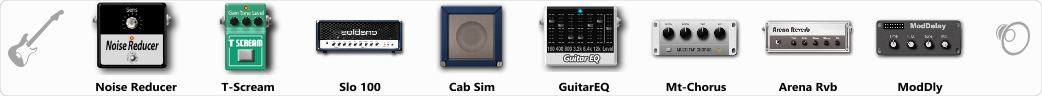Discussion in 'ToneLib-GFX presets' started by bether, Jul 6, 2019.

The best for humbucker, but not onlyEffects chain:Effect: "Noise Reducer" (Dynamics / Filter), active - "yes"
"Sens" = 80
"Mode" = Soft

Effect: "T-Scream" (Overdrive / Distortion), active - "yes"
"Drive" = 23
"Tone" = 79
"Level" = 79

Effect: "Slo 100" (Amp simulators), active - "yes"
"Gain" = 100
"Bass" = 40
"Middle" = 57
"Treble" = 35
"Presence" = 30
"Master" = 69
"Output" = 50
"Level (dB)" = 0

Effect: "Cab Sim" (Cabinets), active - "yes"
"Model" = 4x12" 1960 T75
"Level (dB)" = 0

Effect: "GuitarEQ" (Dynamics / Filter), active - "yes"
"160 Hz" = 0
"400 Hz" = 3
"800 Hz" = 2
"1.6 kHz" = 5
"3.2 kHz" = 1
"6.4 kHz" = 0
"12 kHz" = 0
"Level (dB)" = 0

Effect: "Mt-Chorus" (Modulation / Sfx), active - "yes"
"Speed" = 3.1
"Depth" = 69
"Time" = 3.4
"Mix" = 61

Effect: "Arena Rvb" (Reverb), active - "yes"
"Time" = 7.3
"PreDelay" = 23
"LoDamp" = 18
"HiDamp" = 21
"Mix" = 43

Effect: "ModDly" (Delay), active - "yes"
"Time" = 648
"Feedback" = 39
"Tone" = 70
"Speed" = 1.7
"Mix" = 50

Note: You will need to download and install the ToneLib-GFX software to use the preset.